Definitions of Square Dance Calls and Concepts

Hinge The Lock:
T-Bone \$B\$G\$J\$\$E,@Z\$J(B 2 x 2 \$B\$+\$i!%(BHinge, Lockit \$B\$r\$7\$^\$9!%(BWave \$B\$G=*\$o\$j\$^\$9!%(B

Lock The Hinge:
Wave \$B\$^\$?\$O(B Two-Faced Line \$B\$+\$i!%(BLockit, Hinge \$B\$r\$7\$^\$9!%(BMini-Wave \$B\$N(B Box \$B\$G=*\$o\$j\$^\$9!%(B

\$BN>J}\$H\$b(B 2 \$B%Q!<%H\$N%3!<%k\$G\$9!%(B

Hinge The Lock: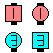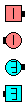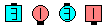Hinge The Lock\$B\$NA0(B Hinge\$B\$N8e(B Lockit\$B\$N8e(B (\$B=*\$o\$j(B)
Lock The Hinge: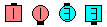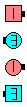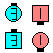Lock The Hinge\$B\$NA0(B Lockit\$B\$N8e(B Hinge\$B\$N8e(B (\$B=*\$o\$j(B)

anything The Lock [C3A]: anything call \$B\$r\$7\$F(B, Lockit \$B\$r\$7\$^\$9!%(B

Lock The anything [C3A]: \$BE,@Z\$J(B formation \$B\$+\$i!%(B Lockit, anything call \$B\$r\$7\$^\$9!%(B

anything The Top [C4]: anything call \$B\$r\$7(B, Fan The Top \$B\$r\$7\$^\$9!%(B

Hinge The Cross Lock [C3B]: Hinge, Cross Lockit \$B\$G\$9!%(B

Cross Lock The Hinge [C3B]: Cross Lockit, Hinge \$B\$G\$9!%(B

\$BCmSnap The Lock [C3A] \$B\$OJL\$N%3!<%k\$G(B anything The Lock \$B\$H\$O4X78\$,\$"\$j\$^\$;\$s!%(B

\$BCross Lockit [C3B].